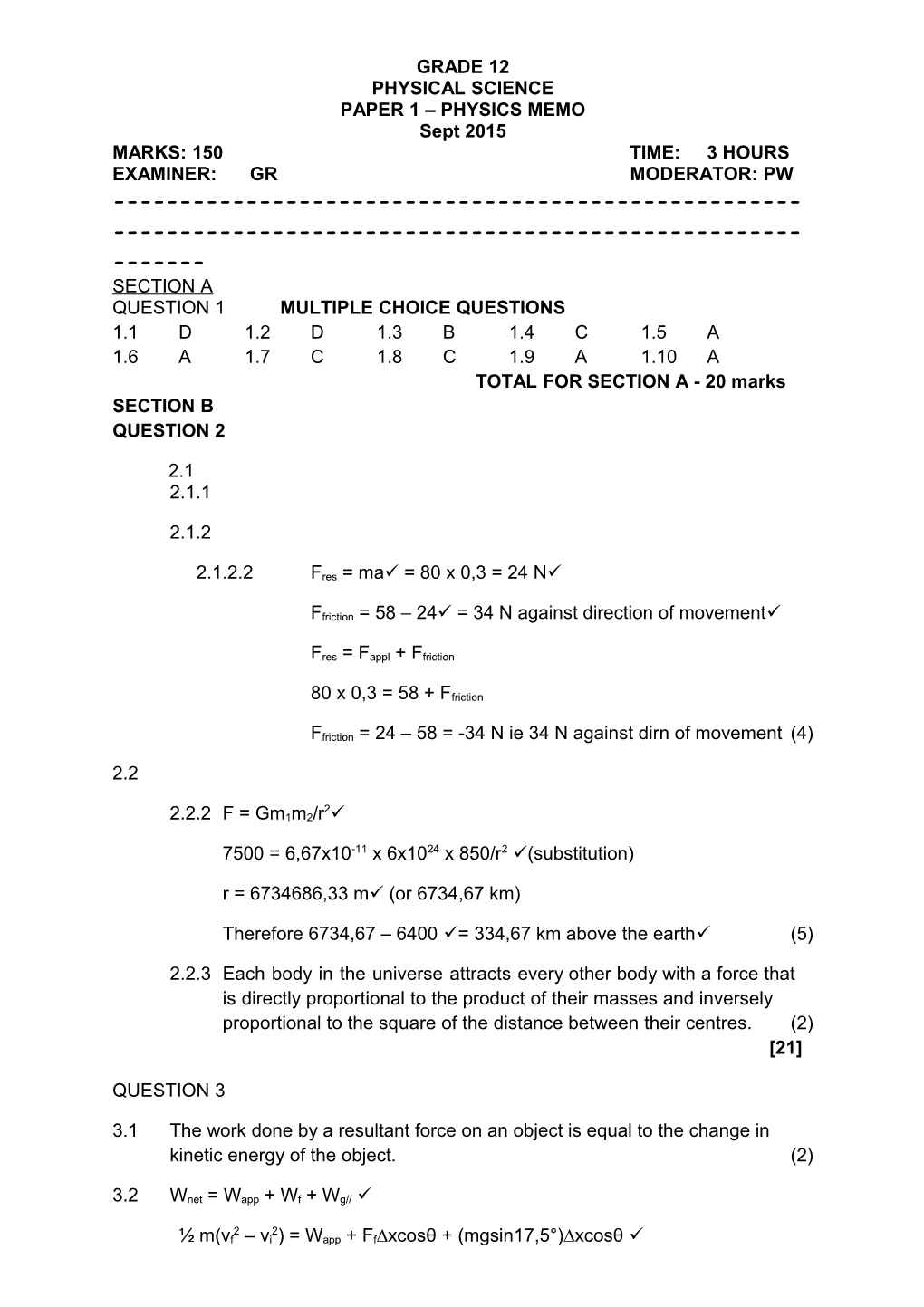# Grade 12 Physical Science Paper 1, Physics September 2015Grade 12 Physical Science – Paper 1, Physics September 2015

Ex: GR

------

PHYSICAL SCIENCE

PAPER 1–PHYSICS MEMO

Sept 2015

MARKS:150TIME:3 HOURS

EXAMINER:GRMODERATOR: PW

------

SECTION A

QUESTION 1MULTIPLE CHOICE QUESTIONS

1.1D1.2D1.3B1.4C1.5A

1.6A1.7C1.8C1.9A1.10A

TOTAL FOR SECTION A - 20 marks

SECTION B

QUESTION 2

2.1

2.1.12.1.22.1.2.2Fres = ma = 80 x 0,3 = 24 N

Ffriction = 58 – 24 = 34 N against direction of movement

Fres = Fappl + Ffriction

80 x 0,3 = 58 + Ffriction

Ffriction = 24 – 58 = -34 N ie 34 N against dirn of movement(4)

2.2

2.2.2F = Gm1m2/r2

7500 = 6,67x10-11 x 6x1024 x 850/r2(substitution)

r = 6734686,33 m (or 6734,67 km)

Therefore 6734,67 – 6400 = 334,67 km above the earth(5)

2.2.3Eachbodyintheuniverseattractsevery otherbodywithaforcethatisdirectlyproportionaltothe productoftheirmassesand inverselyproportionaltothesquareof thedistancebetween their centres. (2)



QUESTION 3

3.1The work done by a resultant force on an object is equal to the change in kinetic energy of the object. (2)

3.2Wnet = Wapp + Wf + Wg//

½ m(vf2 – vi2) = Wapp + Ff∆xcosθ + (mgsin17,5°)∆xcosθ 

½ x 15000 x (122 – 272) = Wapp + 5000 x 130cos180 + 15000x9,8sin17,5° x130 x cos180

Wapp = -4387500 + 650000 + 5746488

= 2,01 x 106 J (7)

3.3Wapp = Fapp∆xcosθ

2,01 x 106= Fapp x 130 x cos0

Fapp = 1,55 x 104 N up slope(4)



QUESTION 4

4.1

4.1.1∆x = vit + ½ gt2

47,3 = 0 + ½ x 9,8 t2(t = )

t = 3,1 s(3)

4.1.2∆x = vit + ½ gt2 (t = 3,1 – 1,4 = 1,7)

47,3 = vi x 1,7 + ½ x 9,8 x 1,72

vi = 19,49 m.s-1(4)

4.2

4.2.1Let forward direction be positive

∆p = m∆v

= 0,08(-6 – 7)

= -1,04 kg.m.s-1 ie 1,04 kg.m.s-1 away from the wall(4)

4.2.2F∆t = m∆v

F x 0,02 = -1,04

F = -52 N ie 52 N away from the wall(3)



QUESTION 5

5.1

5.1.1Thetotalmechanicalenergy (sumofgravitationalpotentialenergy and kinetic energy)inanisolatedsystemremains constant. (2)

5.1.2Mech energy at the bottom = mech energy at the top

(Ek + Ep)bottom = (Ek + Ep)top

½ x 1,16 x v2 + 0 = 0 + 1,16 x 9,8 x 0,27(v = )

v = 2,3 m.s-1(3)

5.1.3In a collision between two objects linear momentum is conserved on both magnitude and direction. (2)

5.1.4Momentum before = momentum after

Σpi = Σpf

m1vi1 +m2vi2 = (m1 + m2)v

0,16u1 + 0 = (1 + 0,16)2,3

u1 = 16,68 m.s-1(4)



QUESTION 6

6.1

6.1.1Doppler effect.(1)

6.1.2

6.1.2.131000/3600 = 8,61 m.s-1(2)

6.1.2.2fL = (v±vL)/(v±vS) x fS

437 = (v + 0)/(v – 8,61) x 426

v = (437/426 x 8,61)/(437/426 – 1)

= 342,05 m.s-1(4)

6.1.2.3As the source approaches the listener, the wavelength of the sound is compressed and as v = fλ, and v is constant, it implies the frequency increases. The opposite is true as the source moves away from the listener.  (Accept calculations using Doppler equation) (4)

6.2

6.2.1Galaxies(1)

6.2.2We would expect to seethat the wavelengths are longer.(1)

6.2.3Red shift(1)

6.2.4They are moving away from us.(1)



QUESTION 7

7.1An electric field is a region of space in which an electric charge experiences a force. (2)

7.2E = kQ/r2= 9x109 x 4x10-9/0,052(conversion)= 14400 N.C-1(4)

7.5FP on Q = mgtan10°

= 0,08x10-3x 9,8tan10°

= 1,38 x 10-4 N(3)

7.6F = QE

Q = 1,38 x 10-4/14400 = 9,58 x 10-9 C(3)

OR: F = kQ1Q2/r2

1,38x10-4 = 9x109 x 4x10-9 x Q2/0,052

Q2 = 9,58 x 10-9 C



QUESTION 8

8.1Light bulbs are identical and resistance in the other branch is double that of the one with A1, therefore half the current through the other branch, so the total current is 4,5 A. (3)

8.2R = V/I = 3,15/1,5 = 2,1 Ω(3)

8.31/Rparallel = 1/rL2 + 1/rL3+4 = 1/2,1 + 1/4,2

Therefore Rparallel = 1,4 Ω

E = I(Rtotal + rint) = 4,5((1,4 +2,1) + 0,8) = 19,35 V(5)

8.4

8.4.1Increase.(1)

8.4.2Decrease(1)

8.4.3Decrease(1)



QUESTION 9

9.1

9.1.1Vrms = Vmax/= 311,3/= 220,12 V(3)

9.1.2Paverage = VrmsIrms

Irms = 100/220,12 = 0,45 A (carry error from 9.1.1)

Irms = Imax/

Therefore Imax = 0,45 x = 0,64 A(5)

9.1.3

9.2

9.2.1The rotating magnet causes a constantly changing linkage between the magnetic flux and the wires of the coil thus inducing a current. (2)

9.2.2AC(1)



QUESTION 10

10.1

10.1.1Minimum energy needed by a photon to eject an electron from a substance. (2)

10.1.2Nuclear content of each metal is different and each metal has a different number of electrons resulting in a different energy required to remove electrons from the metal. (2)

10.1.3Ek = ½ mv2 = ½ x 9,11x10-31 x (4,78x105)2 =1,04 x 10-19 J

E = W0 + Ek (E = hf = hc/λ ; f = c/λ = 1,30x1015 Hz)

6,63x10-34 x 3x108/2,3x10-7 = W0 + 1,04x10-19

W0 = 7,58 x 10-19 J

Therefore the metal is silver(7)



TOTAL FOR SECTION B - 130 marks

TOTAL 150 MARKS

1